# Hypotenuse

Here we will learn about the hypotenuse including how to find the length of the hypotenuse, how to use the hypotenuse to find other missing sides and angles in right angle triangles and how to calculate other values such as the volume or perimeter using the hypotenuse.

There are also hypotenuse worksheets based on Edexcel, AQA and OCR exam questions, along with further guidance on where to go next if you’re still stuck.

## What is the hypotenuse?

The hypotenuse is the longest side of a right angle triangle. It is the side opposite the right angle.

For example,

When we know the vertical height of an isosceles triangle which is perpendicular to the base, we can create two right angle triangles within the isosceles triangle.

### What is the hypotenuse?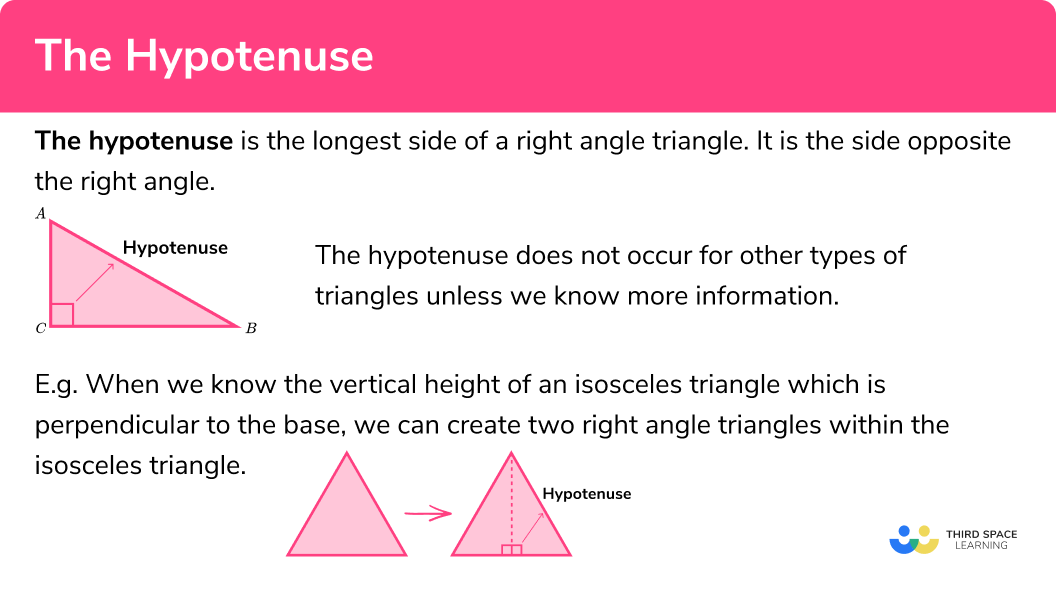## Hypotenuse in 2D and 3D Shapes

We can also calculate diagonals in 2D and 3D shapes by recognising right-angle triangles.

For example,

We can find the diagonal length AC of a parallelogram or the diagonal length AH in the cuboid below.

## Pythagoras or trigonometry?

We need to be able to interpret problems and recognise whether we need to use Pythagoras theorem in 2D, 3D, or one of the three trigonometric ratios.

This flow chart describes the information you need to know about a shape in order to solve the problem.

It is important to recognise that with most of these problems, you may need to use the Pythagorean theorem, or trigonometry, or both within the same question so you must be confident with these topics individually to access this topic fully.

Below is a summary of this information,

NameUsed to find. . . Rule or formula
Pythagoras theorem in 2D The hypotenuse (c) A shorter side (a) \\c=\sqrt{a^{2}+b^{2}} \\a=\sqrt{c^{2}-b^{2}}
Sine functionMissing side: Hypotenuse (H) or Opposite (O) \\H=\frac{O}{\sin(\theta)}\\ \\O=H\times\sin(\theta)
Cosine functionMissing side: Hypotenuse (H) or Adjacent (A) \\H=\frac{A}{\cos(\theta)}\\ \\A=H\times\cos(\theta)
Tangent functionMissing side: Adjacent (A) or Opposite (O) \\A=\frac{O}{\tan(\theta)}\\ \\O=A\times\tan(\theta)
Inverse sine functionMissing angle (\theta)\theta=\sin^{-1}(\frac{O}{H})
Inverse cosine functionMissing angle (\theta)\theta=\cos^{-1}(\frac{A}{H})
Inverse tangent functionMissing angle (\theta)\theta=\tan^{-1}(\frac{O}{A})
Pythagoras theorem in 3DThe diagonal (D)\\D=\sqrt{x^{2}+y^{2}+z^{2}}

## How to calculate the hypotenuse of any right angle triangle

In order to calculate the hypotenuse of any right angle triangle:

1. Determine whether to use Pythagoras theorem or trigonometry.
2. Label the right angle triangle (abc or OAH) and state the formula/rule used.
3. Calculate the missing angle or side.

### Explain how to calculate the hypotenuse of any right angle triangle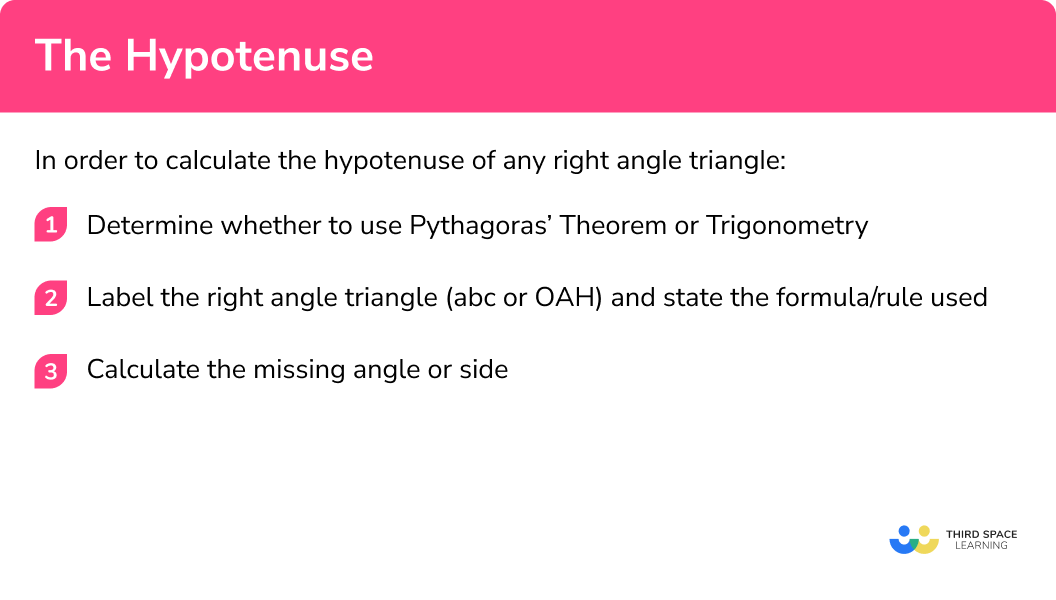## Hypotenuse examples

### Example 1: find the hypotenuse using Pythagoras theorem

Calculate the value of x to 1 decimal place.

1. Determine whether to use Pythagoras theorem or trigonometry.

We know two missing sides of the right angle triangle and no other angles so we can use Pythagoras theorem.

2Label the right angle triangle (abc or OAH) and state the formula/rule used.

As we are using Pythagoras theorem, label the sides a, b, and c (the opposing side to the right angle).

Pythagoras theorem, c=\sqrt{a^{2}+b^{2}} .

3Calculate the missing angle or side.

\begin{aligned} c&=\sqrt{7.3^{2}+12.5^{2}}\\\\ c&=\sqrt{53.29+156.25}\\\\ c&=\sqrt{209.54}\\\\ c&=14.5\mathrm{cm}\quad(1dp) \end{aligned}

### Example 2: find the hypotenuse using trigonometry

Calculate the length of the hypotenuse of a right triangle, x , to 1 decimal place.

Determine whether to use Pythagoras theorem or trigonometry.

Label the right angle triangle (abc or OAH) and state the formula/rule used.

Calculate the missing angle or side.

### Example 3: find the diagonal of a quadrilateral

ABCD is a parallelogram. Calculate the length of the longer diagonal AC to 2 decimal places.

Determine whether to use Pythagoras theorem or trigonometry.

Label the right angle triangle (abc or OAH) and state the formula/rule used.

Calculate the missing angle or side.

### Example 4: find the diagonal of a cuboid using 3D Pythagoras

Calculate the length of the diagonal AH. Write your answer in the form a\sqrt{b}cm where a and b are integers.

Determine whether to use Pythagoras theorem or trigonometry.

Label the right angle triangle (abc or OAH) and state the formula/rule used.

Calculate the missing angle or side.

### Example 5: find the missing length using trigonometry in 3D

ABCDEF is a triangular prism. Calculate the length of the line CF, correct to 3 significant figures..

Determine whether to use Pythagoras theorem or trigonometry.

Label the right angle triangle (abc or OAH) and state the formula/rule used.

Calculate the missing angle or side.

### Example 6: calculating the diagonal of a 3D shape, given the volume

The cube shown has a volume of 648\sqrt{3}\mathrm{cm}^{3} . Calculate the length of the diagonal AH.

Determine whether to use Pythagoras theorem or trigonometry.

Label the right angle triangle (abc or OAH) and state the formula/rule used.

Calculate the missing angle or side.

### Common misconceptions

• Pythagoras theorem or trigonometry

It is a common error to mix up when to use Pythagoras and when to use trigonometry.
Remember in order to use Pythagoras to calculate the unknown side of a right angled triangle we need to know the other two sides.
In order to use trigonometry to calculate the unknown side of a right angled triangle we need to know another side and an angle.

• Incorrectly applying Pythagoras theorem

For example, given the triangle with base 15cm and vertical height 22cm , possible incorrect calculations for the hypotenuse could be:

c=\sqrt{22^{2}-15^{2}}

Or

c=\sqrt{22^{2}\times15^{2}}

The correct calculation is c=\sqrt{22^{2}+15^{2}} = 26.6 (1dp).

• Incorrect trigonometric function

For example, given the triangle below, a possible error would be to incorrectly use the tangent function instead of the cosine function to find the value of x .

### Hypotenuse practice questions

1. Triangle ABC is a right angle triangle. Calculate the value of x .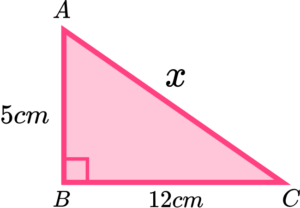10.9cm13cm24.6cm30cmThis is a right angled triangle and we do not know any of the angles so we need to use Pythagoras theorem.

\begin{aligned} c^{2}&=a^{2}+b^{2}\\\\ x^{2}&=5^{2}+12^{2}\\\\ x^{2}&=25+144\\\\ x^{2}&=169\\\\ x&=13\mathrm{cm} \end{aligned}

2. Calculate the value of  \theta to 2 significant figures.36^{\circ}64^{\circ}30^{\circ}12^{\circ}This is a right angled triangle and we are working out the size of an angle so we need to use SOHCAHTOA.

Label the triangle,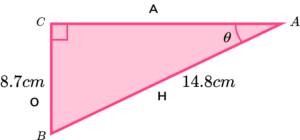We know O and H so use sine.

\begin{aligned} \sin(\theta)&=\frac{O}{H}\\\\ \sin(\theta)&=\frac{8.7}{14.8}\\\\ \theta&= \sin^{-1}(\frac{8.7}{14.8})\\\\ \theta&=36^{\circ} (2sf) \end{aligned}

3. ABCD is a trapezium with diagonal length 10cm .

Calculate \theta , the external angle of the polygon at B , correct to 2 decimal places.0.02^{\circ}71.82^{\circ}5.05^{\circ}53.59^{\circ}First we need to calculate the height of the trapezium. We can do this using the following triangle,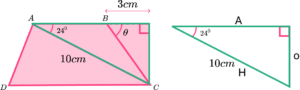The triangle is a right angled triangle and we know an angle so we need to use SOHCAHTOA. We want to work out O and we know H so use sine.

\begin{aligned} O&=H \sin(\theta)\\\\ O&=10 \sin(24)\\\\ O&=4.067366431 \end{aligned}

We can now use the height, along with the information we have been given to find the angle.We know O and A so use tan.

\begin{aligned} \tan(\theta) &= \frac{O}{A}\\\\ \tan(\theta)&=\frac{4.067366431}{3}\\\\ \theta&=\tan^{-1}(\frac{4.067366431}{3})\\\\ \theta&=53.59^{\circ} (2dp) \end{aligned}

4. A swimming pool is the shape of a trapezoidal prism. A sketch of the swimming pool is drawn below.Calculate the longest distance in the pool, AH , correct to 3  significant figures.

78.1ft70.7ft58.3ft67.1ftHere we need to calculate the diagonal length of a 3D shape for which we know the length, width and depth. We can apply Pythagoras theorem in 3D.

\begin{aligned} D^{2}&=x^{2}+y^{2}+z^{2}\\\\ D^{2}&=30^{2}+40^{2}+60^{2}\\\\ D^{2}&=6100\\\\ D&=78.1\mathrm{ft} \end{aligned}

5.  ABC and ADE are two right angle triangles where AD = \frac{2}{3}AC .By calculating the length of AD , find the value of \theta .

35.3^{\circ}45^{\circ}70.5^{\circ}37.8^{\circ}First we need to calculate the length AC,ABC is a right angled triangle and we know an angle so we can use SOHCAHTOA.

We know A and we want to work out O so use tan.

\begin{aligned} O&=A\tan{\theta}\\\\ O&=5\sqrt{3}\tan{60}\\\\ O&=15\mathrm{cm} \end{aligned}

We are told that AD=\frac{2}{3}AC .

Now that we know the length AD , we can use the triangle ADE to work out the required angle.We know O and H so use sin.

\begin{aligned} \sin(\theta)&=\frac{O}{H}\\\\ \sin(\theta)&=\frac{10}{10\sqrt{2}}\\\\ \theta&=\sin^{-1}(\frac{10}{10\sqrt{2}})\\\\ \theta&=45^{\circ} \end{aligned}

6.  The surface area of a cube below is equal to 150cm^2 . Find the diagonal length BE . Give your answer in surd form.25 \sqrt{3} \mathrm{cm}15 \sqrt{2} \mathrm{cm}7.5 \sqrt{2} \mathrm{cm}5 \sqrt{3} \mathrm{cm}This is a cube therefore all 6 faces are the same. If the surface area is 150cm^2 , then the area of each face is 150\div 6=25\mathrm{cm}^{2} and the length of each side is \sqrt{25}=5\mathrm{cm} .

We can then use Pythagoras theorem in 3D to find the length of the diagonal,

\begin{aligned} D^{2}&=x^{2}+y^{2}+z^{2}\\\\ D^{2}&=5^{2}+5^{2}+5^{2}\\\\ D^{2}&=75\\\\ D&=5\sqrt{3} \mathrm{cm} \end{aligned}

### Hypotenuse GCSE exam questions

1.  Circle the correct expression for the perimeter of the kite ABCD .(3 marks)

\begin{aligned} AB&=\frac{5}{sin(30)}\\\\ AB&=10\mathrm{cm} \end{aligned}

(1)

\begin{aligned} BC&=\sqrt{5^{2}+12^{2}}\\\\ BC&=13\mathrm{cm} \end{aligned}

(1)

Total perimeter  = 10+10+13+13 = 46cm

(1)

2.    ABCDE is a square based pyramid with the following details,

• M is the midpoint of BC .
• O is the centre of the square BCDE .
• AM = 42cm.
• BCDE has sides of length 16cm .(a)   Calculate the vertical height of the pyramid, OA .

a\sqrt{b} where a and b are integers.

(b)   Show that AM<AB .

(6 marks)

(a)(1)

OA = \sqrt{42^{2}-8^{2}}

(1)

OA = 10\sqrt{17}cm

(1)

(b)

AB = \sqrt{42^{2}+8^{2}}

(1)

(1)

AM=42cm and AB=42.755cm so AM<AB.

(1)

3.  Two cubes are drawn in the diagram below.Show that  BE = 3.8KN .

(3 marks)

BE=\sqrt{1^{2}+1^{2}+1^{2}} = \sqrt{3}

(1)

KN=\sqrt{3.8^{2}+3.8^{2}+3.8^{2}} = \frac{19\sqrt{3}}{5}

(1)

\frac{19\sqrt{3}}{5}\div{\sqrt{3}}=\frac{19}{5}=3.8

(1)

## Learning checklist

You have now learned how to:

• Use Pythagoras theorem and trigonometric ratios in similar triangles to solve problems involving right-angled triangles
• Apply Pythagoras theorem and trigonometric ratios to find angles and lengths in right-angled triangles (and, where possible, general triangles) in 2 (and 3) dimensional figures

## Still stuck?

Prepare your KS4 students for maths GCSEs success with Third Space Learning. Weekly online one to one GCSE maths revision lessons delivered by expert maths tutors.

Find out more about our GCSE maths tuition programme.